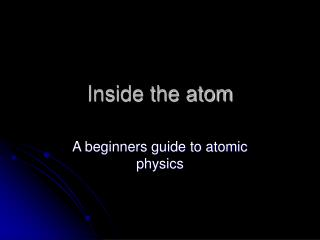# Inside the atom - PowerPoint PPT PresentationDownload PresentationInside the atom

Inside the atomDownload Presentation## Inside the atom

- - - - - - - - - - - - - - - - - - - - - - - - - - - E N D - - - - - - - - - - - - - - - - - - - - - - - - - - -
##### Presentation Transcript

1. Inside the atom A beginners guide to atomic physics

2. Brief History JJ Thomson • Discovered the electron • Where is it? • Plum pudding Model • Rutherford • Gold foil experiment • Positive nuclear core

3. Problems with classical model • Coulomb force could make electrons orbit nucleus like planets, BUT… • Accelerated charge is supposed to emit EM waves…why is atom stable? • Also, why do atoms emit and absorb only discrete frequencies of radiation?

4. BOHR ATOM • Circular electron orbits • Only “stationary” orbits allowed • The energy of each orbit is given by: En= (-13.6 eV) 1/n2(where n is an integer) • Photons of the correct energy are absorbed, “exciting” the atom into a higher energy orbit • Photons are emitted when the electron makes a transition to a lower energy orbit • The energy of the photons corresponds to energy difference of the two orbits DE=hf

5. Bohr atom (continued) Bohr atom...java applet • What wavelength of radiation would excite the hydrogen atom from the ground state (E1) to the third energy level? E1 = -13.6 eV/1 = -13.6 eV E2 = -13.6/9 = -1.5 Ev hc/l = 12.1 eV l = 1243 eV-nm / 12.1 eV = 100 nm

6. What possible wavelengths of light could be emitted when a hydrogen atom “de-excites” from the 4th energy level? -0.82 eV -1.51 eV -3.4 eV -13.6 eV

7. Stationary orbits • Conservation of energy: KE + PE = Etot ½ mv2 – ke2/r = Etotal • Circular motion: F=ma  ke2/r2 = mv2/r  ke2/r = mv2 • Combined: Etotal= mv2 – ½ mv2 = -½ mv2 OR Etotal = ½ ke2/r – ke2/r = -½ ke2/r

8. Stationary orbits (continued) • Which orbits are allowed? • One way of getting the answer is to look at deBroglie wavelength of electrons. • l=h/mv • To “fit” in a particular orbit, 2pr=nl